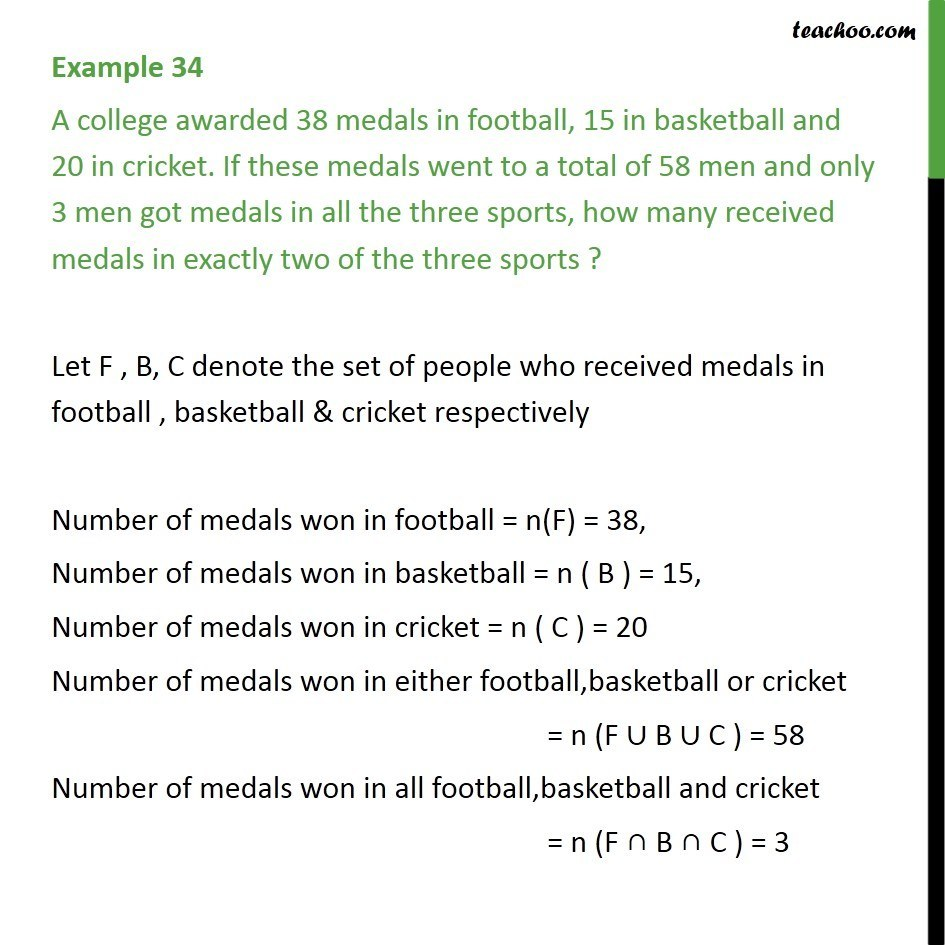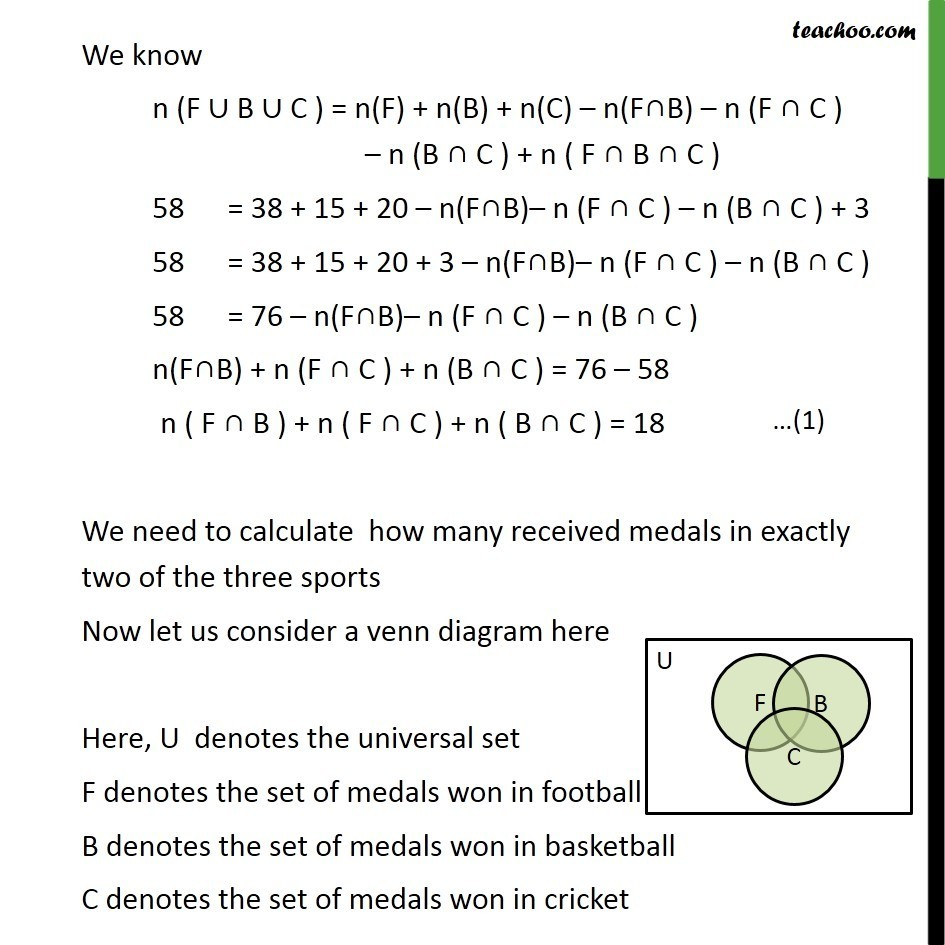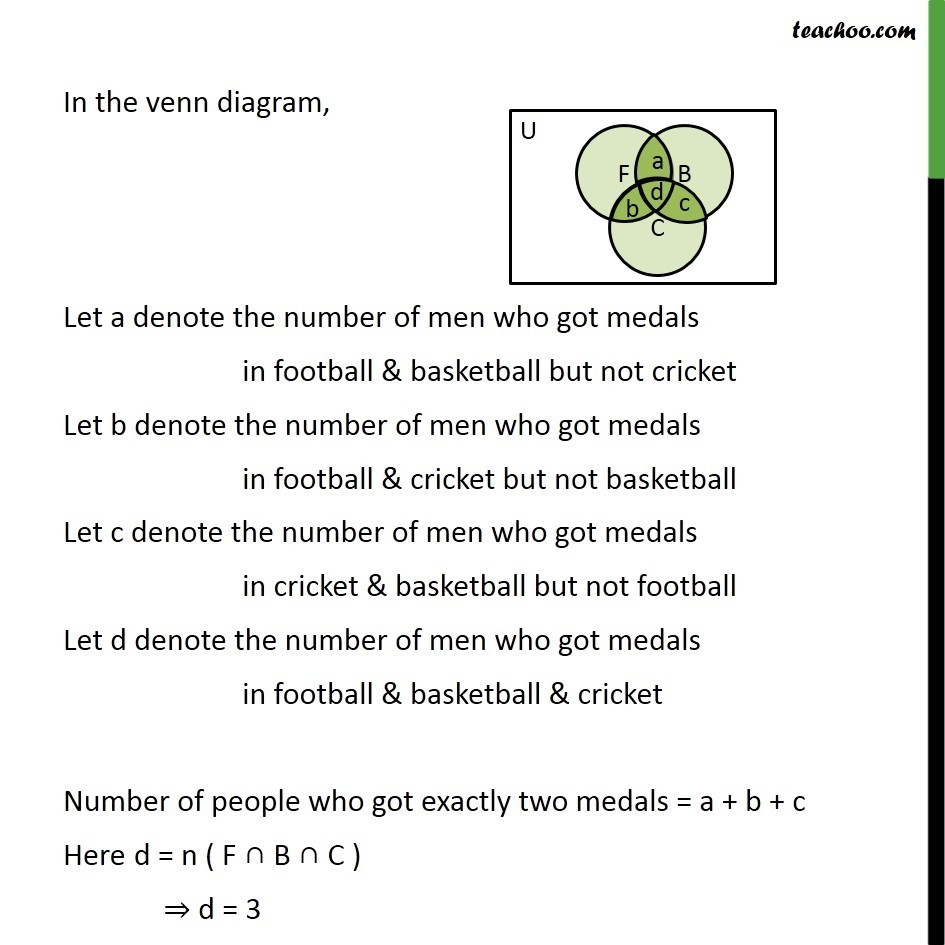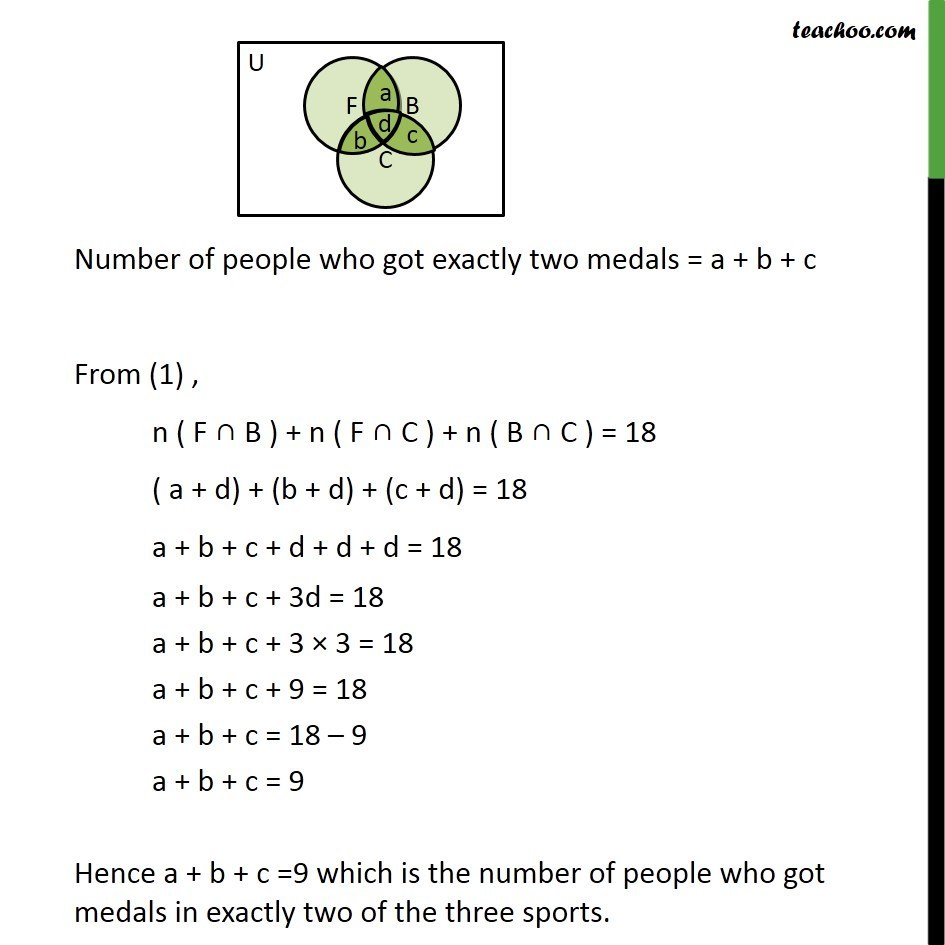1. Chapter 1 Class 11 Sets
2. Serial order wise
3. Examples

Transcript

Example 34 A college awarded 38 medals in football, 15 in basketball and 20 in cricket. If these medals went to a total of 58 men and only 3 men got medals in all the three sports, how many received medals in exactly two of the three sports ? Let F , B, C denote the set of people who received medals in football , basketball & cricket respectively Number of medals won in football = n(F) = 38, Number of medals won in basketball = n ( B ) = 15, Number of medals won in cricket = n ( C ) = 20 Number of medals won in either football,basketball or cricket = n (F B C ) = 58 Number of medals won in all football,basketball and cricket = n (F B C ) = 3 We know n (F B C ) = n(F) + n(B) + n(C) n(F B) n (F C ) n (B C ) + n ( F B C ) 58 = 38 + 15 + 20 n(F B) n (F C ) n (B C ) + 3 58 = 38 + 15 + 20 + 3 n(F B) n (F C ) n (B C ) 58 = 76 n(F B) n (F C ) n (B C ) n(F B) + n (F C ) + n (B C ) = 76 58 n ( F B ) + n ( F C ) + n ( B C ) = 18 We need to calculate how many received medals in exactly two of the three sports Now let us consider a venn diagram here Here, U denotes the universal set F denotes the set of medals won in football B denotes the set of medals won in basketball C denotes the set of medals won in cricket In the venn diagram, Let a denote the number of men who got medals in football & basketball but not cricket Let b denote the number of men who got medals in football & cricket but not basketball Let c denote the number of men who got medals in cricket & basketball but not football Let d denote the number of men who got medals in football & basketball & cricket Number of people who got exactly two medals = a + b + c Here d = n ( F B C ) d = 3 Number of people who got exactly two medals = a + b + c From (1) , n ( F B ) + n ( F C ) + n ( B C ) = 18 ( a + d) + (b + d) + (c + d) = 18 a + b + c + d + d + d = 18 a + b + c + 3d = 18 a + b + c + 3 3 = 18 a + b + c + 9 = 18 a + b + c = 18 9 a + b + c = 9 Hence a + b + c =9 which is the number of people who got medals in exactly two of the three sports.

Examples

Chapter 1 Class 11 Sets
Serial order wise

About the AuthorDavneet Singh
Davneet Singh is a graduate from Indian Institute of Technology, Kanpur. He has been teaching from the past 9 years. He provides courses for Maths and Science at Teachoo.